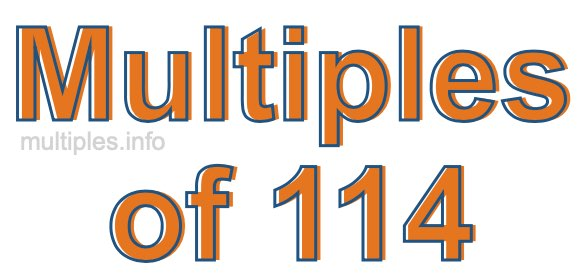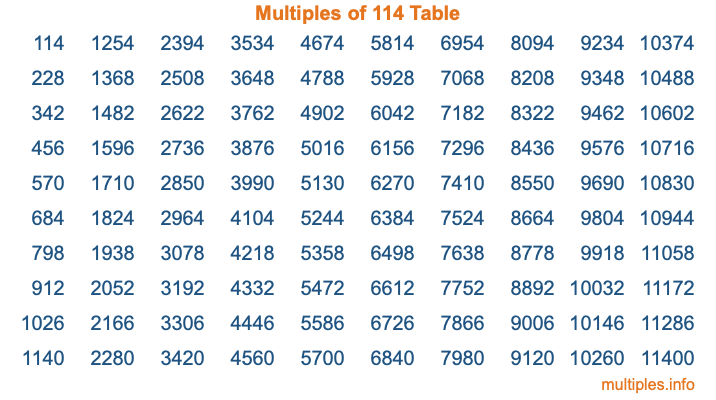Multiples of 114Welcome to the Multiples of 114 page. Here we will first teach you everything you will ever need to know about the multiples of 114, and then give you a study guide summary of everything we taught you to make sure you remember it all. Use this page to look up facts and learn information about the multiples of 114. This page will make you a multiples of one hundred fourteen expert!

Definition of Multiples of 114
Multiples of 114 are all the numbers that when divided by 114 equal an integer. Each of the multiples of 114 are called a multiple. A multiple of 114 is created by multiplying 114 by an integer.

Therefore, to create a list of multiples of 114, you start with 1 multiplied by 114, then 2 multiplied by 114, then 3 multiplied by 114, and so on for as long as you want. Thus, the list of the first five multiples of 114 is 114, 228, 342, 456, and 570. To see a larger list of multiples of 114, see the printable image of Multiples of 114 further down on this page. We also have a category where you can choose any nth multiple of 114.

Multiples of 114 Checker
The Multiples of 114 Checker below checks to see if any number of your choice is a multiple of 114. In other words, it checks to see if there is any number (integer) that when multiplied by 114 will equal your number. To do that, we divide your number by 114. If the the quotient is an integer, then your number is a multiple of 114.

Is  a multiple of 114?

Least Common Multiple of 114 and ...
A Least Common Multiple (LCM) is the lowest multiple that two or more numbers have in common. This is also called the smallest common multiple or lowest common multiple and is useful to know when you are adding our subtracting fractions. Enter one or more numbers below (114 is already entered) to find the LCM.

Check out our LCM Calculator if you need more details about the Least Common Multiple or if you need the LCM for different numbers for adding and subtraction fractions.

nth Multiple of 114
As we stated above, 114 is the first multiple of 114, 228 is the second multiple of 114, 342 is the third multiple of 114, and so on. Enter a number below to find the nth multiple of 114.

th multiple of 114

Multiples of 114 vs Factors of 114
114 is a multiple of 114 and a factor of 114, but that is where the similarities end. All postive multiples of 114 are 114 or greater than 114. All positive factors of 114 are 114 or less than 114.

Below is the beginning list of multiples of 114 and the factors of 114 so you can compare:

Multiples of 114: 114, 228, 342, 456, 570, etc.

Factors of 114: 1, 2, 3, 6, 19, 38, 57, 114

As you can see, the multiples of 114 are all the numbers that you can divide by 114 to get a whole number. The factors of 114, on the other hand, are all the whole numbers that you can multiply by another whole number to get 114.

It's also interesting to note that if a number (x) is a factor of 114, then 114 will also be a multiple of that number (x).

Multiples of 114 vs Divisors of 114
The divisors of 114 are all the integers that 114 can be divided by evenly. Below is a list of the divisors of 114.

Divisors of 114: 1, 2, 3, 6, 19, 38, 57, 114

The interesting thing to note here is that if you take any multiple of 114 and divide it by a divisor of 114, you will see that the quotient is an integer.

Multiples of 114 Table
Below is an image of the first 100 multiples of 114 in a table. The table is in chronological order, column by column. The first column has the first ten multiples of 114, the second column has the next ten multiples of 114, and so on.The Multiples of 114 Table is also referred to as the 114 Times Table or Times Table of 114. You are welcome to print out our table for your studies.

Negative Multiples of 114
Although not often discussed or needed in math, it is worth mentioning that you can make a list of negative multiples of 114 by multiplying 114 by -1, then by -2, then by -3, and so on, to get the following list of negative multiples of 114:

-114, -228, -342, -456, -570, etc.

Multiples of 114 Summary
Below is a summary of important Multiples of 114 facts that we have discussed on this page. To retain the knowledge on this page, we recommend that you read through the summary and explain to yourself or a study partner why they hold true.

There are an infinite number of multiples of 114.

A multiple of 114 divided by 114 will equal a whole number.

114 divided by a factor of 114 equals a divisor of 114.

The nth multiple of 114 is n times 114.

The largest factor of 114 is equal to the first positive multiple of 114.

114 is a multiple of every factor of 114.

114 is a multiple of 114.

A multiple of 114 divided by a divisor of 114 equals an integer.

114 divided by a divisor of 114 equals a factor of 114.

Any integer times 114 will equal a multiple of 114.

Multiples of a Number
Here you can get the multiples of another number, all with the same attention to detail as we did for multiples of 114 on this page.

Multiples of
Multiples of 115
Did you find our page about multiples of one hundred fourteen educational? Do you want more knowledge? Check out the multiples of the next number on our list!